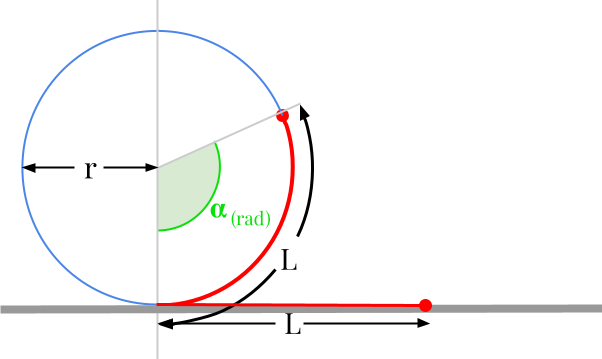Question #813

# What is the formula to convert radians [rad] to meters [m]?

Merged questions

You can't actually convert radians to meters. Radian is a measure of angle, while meters is a unit of distance. There is an homogeneity issue between the two quantities.However, there are many mechanical systems that transform rotary motion into linear motion. The most obvious system is the wheel: the rotation of the wheel moves the vehicle forward and vice versa. In this case, the formula governing such a system and allowing to transform a rotation of an angle expressed in radians into a displacement given in meters is given by:

﻿﻿

﻿﻿

with :

• ﻿﻿ the distance or length expressed in metres [m]
• ﻿﻿ is the radius expressed in metres [m]